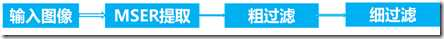# matlab实现MSER（最大极值稳定区域）来进行文本定位

1. 基于连通域的方式

连通域分析是在场景文本图像二值化后进行的，所以开始的研究集中在场景文本图像的预处理、增强和二值化上。基于连通域的方式很少需要在多尺度上进行操作，所以运算时间较快，但存在需要大量的先验信息来滤除文本区域的弊端。

2. 基于学习的方式

基于学习的方式一般流程是先将图像分割成一个个窗口，提取窗口中图像的特征，然后利用一个训练好的分类器来将窗口分成文本和非文本，最后将文本区域连成一个文本行。基于学习的方式，计算量大，一般都在多尺度上处理，而且需要先准备好训练的数据来训练分类器。

3. 连通域和学习结合的方式

这一方式一般都是分为两个阶段，阶段一是提取候选的连通区域，这个阶段一般采用的是连通域分析的方法。阶段二是文本区域和非文本区域的分类，这一阶段一般是采用分类器的方式实现的。两者结合的方式虽然没有众多的参数设置，但还是无法摆脱训练数据的限制。

最大极值稳定区域是由Matas等人提出的一种仿射特征区域提取算法。其提取的区域内部灰度几乎不变但是和背景的对比十分强烈，并且该区域能够在多重阈值下保持形状不变。一般文本内部的灰度变化都比较小，而文本和背景的灰度对比度则比较大，符合最大极值稳定区域的特性，因此利用这一特性可以提取颜色聚类无法得到的部分连通域。

1.处理流程首先是输入一幅图像，想进行必要的预处理如灰度化，然后提取MSER区域（这里直接利用的是matlab自带的函数detectMSERFeature），然后将得到的区域转换成二值图像（主要是利用取得区域的坐标信息）。对得到的MSER区域二值图像进行连通域分析，先粗过滤一些明显不符合字符的区域，然后对过滤后的图像进行闭运算。闭运算之后再进行一次细滤除，最后得到包围文本区域的包围盒。先看几组效果，左边是原图，右边是定位后的图，绿色线画出来的区域，不是很明显。图是比较简单，说明这个方法还是比较初级的，存在比较多的经验阈值。

2、源代码

整个主函数

```%% 读取图片
[filename,pathname]=uigetfile(‘*.*‘,‘choose a picture‘);
path = [pathname filename];
figure;imshow(colorImage);
%% mser区域提取
grayImage = rgb2gray(colorImage);
mserRegions = detectMSERFeatures(grayImage);
mserRegionsPixels = vertcat(cell2mat(mserRegions.PixelList));

%%  把mser区域的坐标系数取出来，然后将相应系数的地方赋值为真。取出mser区域。

%% 粗滤除
figure;imshow(colorImage);
wi= median(cwidth(:))/2;
se1=strel(‘line‘,wi,0);
p_image_dilate= imclose(p_image,se1);

%% 细滤除
[rec_word,img_color,img_bw]=f_conComp_analysis(p_image_dilate,colorImage,p_image);```

```function [p_image,cwidth] =conComp_analysis(bwimg)

[x,y]=size(bwimg);
cwidth=[];
whole=x*y;
connComp = bwconncomp(bwimg); % Find connected components
threefeature = regionprops(connComp,‘Area‘,‘BoundingBox‘,‘Centroid‘  );
broder=[threefeature.BoundingBox];%[x y width height]字符的区域
area=[threefeature.Area];%区域面积
centre=[threefeature.Centroid];
%%
for i=1:connComp.NumObjects
leftx=broder((i-1)*4+1);
lefty=broder((i-1)*4+2);
width=broder((i-1)*4+3);
height=broder((i-1)*4+4);
cenx=floor(centre((i-1)*2+1));
ceny=floor(centre((i-1)*2+2));

if area(i)<80||area(i)>0.3*whole
bwimg(connComp.PixelIdxList{i})=0;
elseif width/height<0.1||width/height>2
bwimg(connComp.PixelIdxList{i})=0;
else
cwidth=[cwidth,width];
rectangle(‘Position‘,[leftx,lefty,width,height], ‘EdgeColor‘,‘g‘);
end
end
p_image=bwimg;```

其中的f_conComp_analysis，这个函数就不细讲了，跟上面的类似。同时保存了彩色和灰度的文本词图像，为识别做进一步准备。

```function [rec,seg_img_color,seg_img_bw] =f_conComp_analysis(P_image,colorImg,p_img)
[x,y]=size(P_image);
whole=x*y;
j=1;
rec=[];
connComp = bwconncomp(P_image); % Find connected components
threefeature = regionprops(connComp,‘Area‘,‘BoundingBox‘);
broder=[threefeature.BoundingBox];%[x y width height]字符的区域
area=[threefeature.Area];%区域面积
%%
for i=1:connComp.NumObjects
leftx=floor(broder((i-1)*4+1));
lefty=floor(broder((i-1)*4+2));
width=broder((i-1)*4+3);
height=broder((i-1)*4+4);

%    data=grayimg_reserve(lefty:lefty+height-1,leftx:leftx+width-1);
%    stda(i,:)=statxture(data);
if area(i)<500||area(i)>whole*0.4
P_image(connComp.PixelIdxList{i})=0;
elseif width/height<2
P_image(connComp.PixelIdxList{i})=0;
%     elseif stda(i,4)<0
%     P_image(connComp.PixelIdxList{i})=0;
else
rect=[leftx,lefty,width,height];
rec=[rec;rect];
rectangle(‘Position‘,[leftx,lefty,width,height], ‘EdgeColor‘,‘g‘);
seg_img_color{j}=colorImg(lefty+1:lefty+height,leftx+1:leftx+width,:); % +1 避免索引为0
seg_img_bw{j}=p_img(lefty+1:lefty+height,leftx+1:leftx+width);
j=j+1;
%         zone{1,j}.data=grayimg_reserve(lefty:lefty+height-1,leftx:leftx+width-1);
%         zone{1,j}.location=[leftx,lefty,width,height];
%         zone{1,j}.label=j;
%         j=j+1;
end
end
pp_image=P_image;```

matlab实现MSER（最大极值稳定区域）来进行文本定位

(3)
(2)

2016-05-02 17:21:07

(2)
(8)
1条